Disclosure: This post may contain affiliate links, meaning when you click the links and make a purchase, we receive a commission.

# Multiple Regression Analysis with Excel

Simple regression analysis is commonly used to estimate the relationship between two variables, for example, the relationship between crop yields and rainfalls or the relationship between the taste of bread and oven temperature.  However, we need to investigate the relationship between a dependent variable and two or more independent variables more often than not. For example, a real estate agent may want to know whether and how measures such as the size of the house, the number of bedrooms and the average income of neighborhood relate to the price for which a house is sold. This kind of problem can be solved by applying multiple regression analysis. And this article will give you a summary on how to use do multiple regression analysis using Excel.

## Problem

Suppose that we took 5 randomly selected salespeople and collected the information as shown in below table.  Whether education or motivation has an impact on annual sales or not?

 Highest Year of School Completed Motivation as Measured by Higgins Motivation Scale Annual Sales in Dollars 12 32 \$350,000 14 35 \$399,765 15 45 \$429,000 16 50 \$435,000 18 65 \$433,000

## Equation

Generally, multiple regression analysis assumes that there is a linear relationship between the dependent variable (y) and independent variables (x1, x2, x3 … xn). And this kind of linear relationship can be described using the following formula:

Y = constant + β1*x1 + β2*x2+…+ βn*xn

Here are the explanations for constants and coefficients:

 Y The predicted value of Y Constant The Y-intercept β1 The change in Y each 1 increment change in x1 β2 The change in Y each 1 increment change in x2 … … βn The change in Y each 1 increment change in xn

Constant and β1, β2… βn can be calculated based on available sample data. After you get values of constant, β1, β2… βn, you can use them to make the predictions.

As for our problem, there are only two factors in which we have an interest. Therefore, the equation will be:

Annual sales = constant + β1*(Highest Year of School Completed) + β2*(Motivation as Measured by Higgins Motivation Scale)

## Set Up Model

Annual sales, highest year of school completed and Motivation was entered into column A, column B, and column C as shown in Figure 1. It is better to always put the dependent variable (Annual sales here) before independent variables.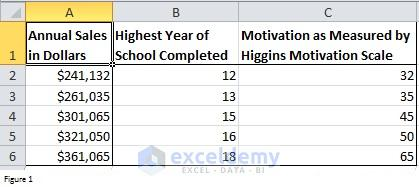Figure 1

Excel offers us Data Analysis feature which can return values of constant and coefficients. But before using this feature, you need to download Analysis ToolPak. Here is how you can install it.

Click on the File tab -> Options and then click on Add-Ins in Excel Options dialog box. Click on Go button at the bottom of Excel Options dialog box to open Add-Ins dialog box. In the Add-Ins dialog box, select Analysis TookPak checkbox and then click on Ok.

Now if you click on Data tab, you will see Data Analysis appears in the Analysis group (right panel).

## Multiple Regression Analysis

Click on Data Analysis in the Analysis group on the Data tab. Select Regression In the prompted Data Analysis dialog box. You can also do other statistical analysis such as t-test, ANOVA and so on.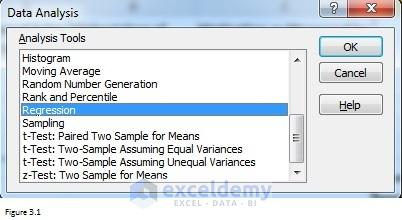Figure 3.1

A Regression dialog box will be prompted after you select Regression. Fill the dialog box as shown in Figure 3.2.

Input Y Range contains the dependent variable and data while the Input X Range contains independent variables and data. Here I have to remind you that independent variables should be in adjacent columns. And the maximum number of independent variables is 15.

Read More: How to Find Correlation between Two Variables in Excel

Since range A1: C1 includes variable labels and therefore Labels check box should be selected. In fact, I recommend you to include labels every time when you fill Input Y Range and Input X Range. These labels are helpful when you review summary reports returned by Excel.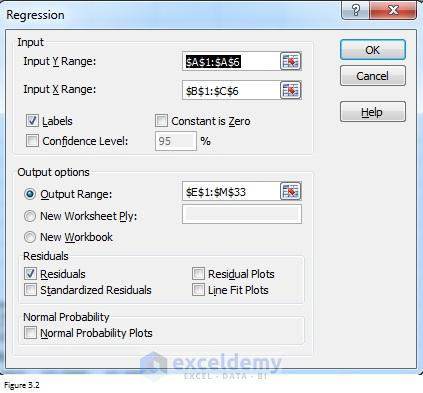Figure 3.2

By selecting the Residuals check box, you can enable Excel to list residuals for each observation. Look at Figure 1, there are 5 observations in total and you will get 5 residuals. Residual is something that’s left when you subtract the predicted value from the observed value. Standardized residual is the residual divided by its standard deviation.

You can also select Residual Plot checkbox which can enable Excel to return residual plots. The number of residual plots equals to the number of independent variables. A residual plot is a graph that shows the residuals on the Y axis and independent variables on the x-axis. Randomly dispersed points around x-axis in a residual plot imply that the linear regression model is appropriate. For example, Figure 3.3 shows three typical patterns of residual plots. Only the one in the left panel indicates that it is a good fit for a linear model. The other two patterns suggest a better fit for a non-linear model.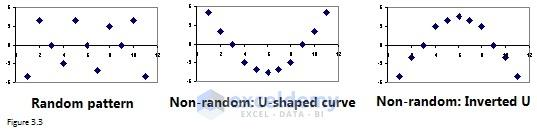Figure 3.3

Excel will return a fitted line plot if you select Line Fit Plots check box. A fitted line plot can plot the relationship between one dependent variable and one independent variable. In other words, Excel will return you the same number of fitted line plots with that of the independent variable. For example, you will get 2 fitted line plots for our problem.

## Results

After you click on the Ok button, Excel will return a summary report as below. Cells highlighted in green and yellow are the most important part on which you should pay your attention.Figure 3.4

The higher R-square (cell F5), the tight relationship exists between dependent variables and independent variables. And coefficients (range F17: F19) in the third table returned you the values of constants and coefficients. The equation should be Annual sales = 1589.2 + 19928.3*(Highest Year of School Completed) + 11.9*(Motivation as Measured by Higgins Motivation Scale).

However, to see if the results are reliable, you also need to check p-values highlighted in yellow. Only if p-value in cell J12 is less than 0.05, the whole regression equation is reliable. But you also need to check p-values in range I17: I19 to see if constant and independent variables are useful for prediction of the dependent variable. For our problem, it is better for us to discard motivation when considering independent variables.

Read More: How to Create One-Variable Data Table in Excel 2013 – [What If Analysis]

## Remove Motivation from independent variables

After deleting Motivation as the independent variable, I applied the same approach and did a simple regression analysis. You can see that all of the values are less than 0.05 now. The final equation should be:

Annual sales = 1167.8 + 19993.3*(Highest Year of School Completed)

## Note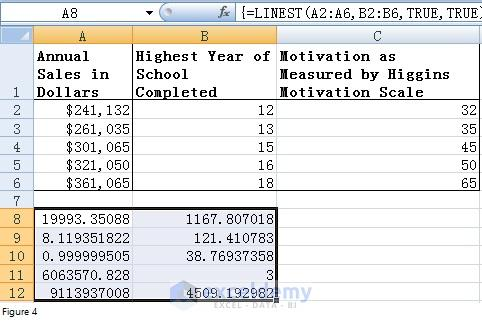Figure 4

Besides Add-Ins tool, you can also use LINEST function to do multiple regression analysis. LINEST function is an array function which can return the result in either one cell or a range of cells. First of all, select range A8:B12 and then enter formula “=LINEST (A2:A6, B2:B6, TRUE, TRUE)” into the first cell of this range (A8). After you press CTRL + SHIFT +ENTER, Excel will return results as below. By comparing against Figure 3.4, you can see that 19993.3 is the coefficient of Highest year of school completed while 1167.8 is constant. Anyway, I recommend you to use Add-Ins tool. It is much easier.

Reverse What-If Analysis in Excel

How to Use Wildcards in Excel?

1. Reply